ElectromagnetismElectricity ·Magnetism
Electrodynamics
Lorentz force law · EMF · Electromagnetic induction · Faraday’s law · Displacement current · Maxwell’s equations · EM field · Electromagnetic radiation · Liénard-Wiechert Potentials · Maxwell tensor · Eddy current ·

Electromagnetic radiation (EM radiation or EMR) takes the form of self-propagating waves in a vacuum or in matter. EM radiation has both electric and magnetic field components, which oscillate in phase perpendicular to each other and to the direction of energy propagation. It carries energy and momentum, which may be imparted when it interacts with matter.

Electromagnetic radiation is subdivided into various types, according to the frequency of the wave. These types include (in order of increasing frequency): radio waves, microwaves, terahertz radiation, infrared radiation, visible light, ultraviolet radiation, X-rays, and gamma rays. Of these, radio waves have the longest wavelengths and gamma rays have the shortest. A small window of frequencies, called the visible spectrum or light, is perceived by the eye of various organisms, with variations of the limits of this narrow spectrum.

## Physics

### Theory

Electromagnetic waves were first postulated by James Clerk Maxwell and subsequently confirmed by Heinrich Hertz. Maxwell derived a wave form of the electric and magnetic equations, revealing the wave-like nature of electric and magnetic fields, and their symmetry. Because the speed of EM waves predicted by the wave equation coincided with the measured speed of light, Maxwell concluded that light itself is an EM wave.

According to Maxwell's equations, a time-varying electric field generates a magnetic field and vice versa. Therefore, as an oscillating electric field generates an oscillating magnetic field, the magnetic field in turn generates an oscillating electric field, and so on. These oscillating fields together form an electromagnetic wave.

A quantum theory of the interaction between electromagnetic radiation and matter such as electrons is described by the theory of quantum electrodynamics.

### Properties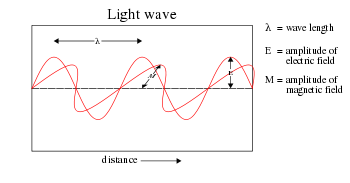Electromagnetic waves can be imagined as a self-propagating transverse oscillating wave of electric and magnetic fields. This diagram shows a plane linearly polarized wave propagating from right to left. The electric field is in a vertical plane, the magnetic field in a horizontal plane.

Electric and magnetic fields do obey the properties of superposition, so fields due to particular particles or time-varying electric or magnetic fields contribute to the fields due to other causes. (As these fields are vector fields, all magnetic and electric field vectors add together according to vector addition.) These properties cause various phenomena including refraction and diffraction. For instance, a traveling EM wave incident on an atomic structure induces oscillation in the atoms, thereby causing them to emit their own EM waves. These emissions then alter the impinging wave through interference.

Since light is an oscillation, it is not affected by traveling through static electric or magnetic fields in a linear medium such as a vacuum. In nonlinear media such as some crystals, however, interactions can occur between light and static electric and magnetic fields - these interactions include the Faraday effect and the Kerr effect.

In refraction, a wave crossing from one medium to another of different density alters its speed and direction upon entering the new medium. The ratio of the refractive indices of the media determines the degree of refraction, and is summarized by Snell's law. Light disperses into a visible spectrum as light is shone through a prism because of the wavelength dependant refractive index of the prism material (Dispersion).

The physics of electromagnetic radiation is electrodynamics, a subfield of electromagnetism.

EM radiation exhibits both wave properties and particle properties at the same time (see wave-particle duality). The wave characteristics are more apparent when EM radiation is measured over relatively large timescales and over large distances, and the particle characteristics are more evident when measuring small distances and timescales. Both characteristics have been confirmed in a large number of experiments.

There are experiments in which the wave and particle natures of electromagnetic waves appear in the same experiment, such as the diffraction of a single photon. When a single photon is sent through two slits, it passes through both of them interfering with itself, as waves do, yet is detected by a photomultiplier or other sensitive detector only once. Similar self-interference is observed when a single photon is sent into a Michelson interferometer or other interferometers.

### Wave model

An important aspect of the nature of light is frequency. The frequency of a wave is its rate of oscillation and is measured in hertz, the SI unit of frequency, where one hertz is equal to one oscillation per second. Light usually has a spectrum of frequencies which sum together to form the resultant wave. Different frequencies undergo different angles of refraction.

A wave consists of successive troughs and crests, and the distance between two adjacent crests or troughs is called the wavelength. Waves of the electromagnetic spectrum vary in size, from very long radio waves the size of buildings to very short gamma rays smaller than atom nuclei. Frequency is inversely proportional to wavelength, according to the equation:$\displaystyle v=f\lambda$

where v is the speed of the wave (c in a vacuum, or less in other media), f is the frequency and λ is the wavelength. As waves cross boundaries between different media, their speeds change but their frequencies remain constant.

Interference is the superposition of two or more waves resulting in a new wave pattern. If the fields have components in the same direction, they constructively interfere, while opposite directions cause destructive interference.

The energy in electromagnetic waves is sometimes called radiant energy.

### Particle model

Because energy of an EM wave is quantized, in the particle model of EM radiation, a wave consists of discrete packets of energy, or quanta, called photons. The frequency of the wave is proportional to the magnitude of the particle's energy. Moreover, because photons are emitted and absorbed by charged particles, they act as transporters of energy. The energy per photon can be calculated by Planck's equation: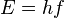$\displaystyle E=hf$

where E is the energy, h is Planck's constant, and f is frequency. This photon-energy expression is a particular case of the energy levels of the more general electromagnetic oscillator whose average energy, which is used to obtain Planck's radiation law, can be shown to differ sharply from that predicted by the equipartition principle at low temperature, thereby establishes a failure of equipartition due to quantum effects at low temperature.

As a photon is absorbed by an atom, it excites an electron, elevating it to a higher energy level. If the energy is great enough, so that the electron jumps to a high enough energy level, it may escape the positive pull of the nucleus and be liberated from the atom in a process called photoionisation. Conversely, an electron that descends to a lower energy level in an atom emits a photon of light equal to the energy difference. Since the energy levels of electrons in atoms are discrete, each element emits and absorbs its own characteristic frequencies.

Together, these effects explain the absorption spectra of light. The dark bands in the spectrum are due to the atoms in the intervening medium absorbing different frequencies of the light. The composition of the medium through which the light travels determines the nature of the absorption spectrum. For instance, dark bands in the light emitted by a distant star are due to the atoms in the star's atmosphere. These bands correspond to the allowed energy levels in the atoms. A similar phenomenon occurs for emission. As the electrons descend to lower energy levels, a spectrum is emitted that represents the jumps between the energy levels of the electrons. This is manifested in the emission spectrum of nebulae. Today, scientists use this phenomenon to observe what elements a certain star is composed of. It is also used in the determination of the distance of a star, using the so-called red shift.

### Speed of propagation

Any electric charge which accelerates, or any changing magnetic field, produces electromagnetic radiation. Electromagnetic information about the charge travels at the speed of light. Accurate treatment thus incorporates a concept known as retarded time (as opposed to advanced time, which is unphysical in light of causality), which adds to the expressions for the electrodynamic electric field and magnetic field. These extra terms are responsible for electromagnetic radiation. When any wire (or other conducting object such as an antenna) conducts alternating current, electromagnetic radiation is propagated at the same frequency as the electric current. Depending on the circumstances, it may behave as a wave or as particles. As a wave, it is characterized by a velocity (the speed of light), wavelength, and frequency. When considered as particles, they are known as photons, and each has an energy related to the frequency of the wave given by Planck's relation E = hν, where E is the energy of the photon, h = 6.626 × 10-34 J•s is Planck's constant, and ν is the frequency of the wave.

One rule is always obeyed regardless of the circumstances: EM radiation in a vacuum always travels at the speed of light, relative to the observer, regardless of the observer's velocity. (This observation led to Albert Einstein's development of the theory of special relativity.)

In a medium (other than vacuum), velocity factor or refractive index are considered, depending on frequency and application. Both of these are ratios of the speed in a medium to speed in a vacuum.

## Electromagnetic spectrum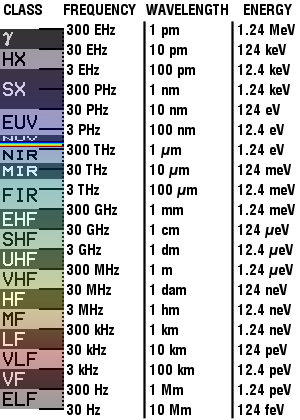Legend:
_ = Gamma rays
HX = Hard X-rays
SX = Soft X-Rays
EUV = Extreme ultraviolet
NUV = Near ultraviolet
Visible light
NIR = Near infrared
MIR = Moderate infrared
FIR = Far infrared

EHF = Extremely high frequency (Microwaves)
SHF = Super high frequency (Microwaves)
UHF = Ultrahigh frequency (Microwaves)
VHF = Very high frequency
HF = High frequency
MF = Medium frequency
LF = Low frequency
VLF = Very low frequency
VF = Voice frequency
ELF = Extremely low frequency

Generally, EM radiation is classified by wavelength into electrical energy, radio, microwave, infrared, the visible region we perceive as light, ultraviolet, X-rays and gamma rays.

The behavior of EM radiation depends on its wavelength. Higher frequencies have shorter wavelengths, and lower frequencies have longer wavelengths. When EM radiation interacts with single atoms and molecules, its behavior depends on the amount of energy per quantum it carries. Spectroscopy can detect a much wider region of the EM spectrum than the visible range of 400 nm to 700 nm. A common laboratory spectroscope can detect wavelengths from 2 nm to 2500 nm. Detailed information about the physical properties of objects, gases, or even stars can be obtained from this type of device. It is widely used in astrophysics. For example, hydrogen atoms emit radio waves of wavelength 21.12 cm.

### Light

Main article: Light

EM radiation with a wavelength between approximately 400 nm and 700 nm is detected by the human eye and perceived as visible light. Other wavelengths, especially nearby infrared (longer than 700 nm) and ultraviolet (shorter than 400 nm) are also sometimes referred to as light, especially when the visibility to humans is not relevant.

If radiation having a frequency in the visible region of the EM spectrum reflects off of an object, say, a bowl of fruit, and then strikes our eyes, this results in our visual perception of the scene. Our brain's visual system processes the multitude of reflected frequencies into different shades and hues, and through this not-entirely-understood psychophysical phenomenon, most people perceive a bowl of fruit.

At most wavelengths, however, the information carried by electromagnetic radiation is not directly detected by human senses. Natural sources produce EM radiation across the spectrum, and our technology can also manipulate a broad range of wavelengths. Optical fiber transmits light which, although not suitable for direct viewing, can carry data that can be translated into sound or an image. The coding used in such data is similar to that used with radio waves.

Radio waves can be made to carry information by varying a combination of the amplitude, frequency and phase of the wave within a frequency band.

When EM radiation impinges upon a conductor, it couples to the conductor, travels along it, and induces an electric current on the surface of that conductor by exciting the electrons of the conducting material. This effect (the skin effect) is used in antennas. EM radiation may also cause certain molecules to absorb energy and thus to heat up; this is exploited in microwave ovens.

## Derivation

Electromagnetic waves as a general phenomenon were predicted by the classical laws of electricity and magnetism, known as Maxwell's equations. If you inspect Maxwell's equations without sources (charges or currents) then you will find that, along with the possibility of nothing happening, the theory will also admit nontrivial solutions of changing electric and magnetic fields. Beginning with Maxwell's equations for free space: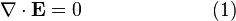$\nabla \cdot \mathbf{E} = 0 \qquad \qquad \qquad \ \ (1)$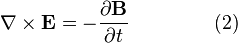$\nabla \times \mathbf{E} = -\frac{\partial \mathbf{B}}{\partial t} \qquad \qquad \ (2)$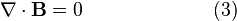$\nabla \cdot \mathbf{B} = 0 \qquad \qquad \qquad \ \ (3)$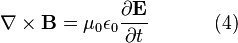$\nabla \times \mathbf{B} = \mu_0 \epsilon_0 \frac{\partial \mathbf{E}}{\partial t} \qquad \quad \ (4)$
where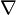$\nabla$ is a vector differential operator (see Del).

One solution,$\mathbf{E}=\mathbf{B}=\mathbf{0}$,

is trivial.

To see the more interesting one, we utilize vector identities, which work for any vector, as follows: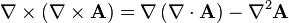$\nabla \times \left( \nabla \times \mathbf{A} \right) = \nabla \left( \nabla \cdot \mathbf{A} \right) - \nabla^2 \mathbf{A}$

To see how we can use this take the curl of equation (2):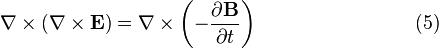$\nabla \times \left(\nabla \times \mathbf{E} \right) = \nabla \times \left(-\frac{\partial \mathbf{B}}{\partial t} \right) \qquad \qquad \qquad \quad \ \ \ (5) \,$

Evaluating the left hand side: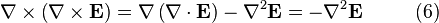$\nabla \times \left(\nabla \times \mathbf{E} \right) = \nabla\left(\nabla \cdot \mathbf{E} \right) - \nabla^2 \mathbf{E} = - \nabla^2 \mathbf{E} \qquad \ \ (6) \,$
where we simplified the above by using equation (1).

Evaluate the right hand side: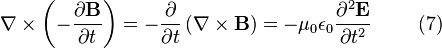$\nabla \times \left(-\frac{\partial \mathbf{B}}{\partial t} \right) = -\frac{\partial}{\partial t} \left( \nabla \times \mathbf{B} \right) = -\mu_0 \epsilon_0 \frac{\partial^2 \mathbf{E}}{\partial t^2} \quad \ \ \ \ (7)$

Equations (6) and (7) are equal, so this results in a vector-valued differential equation for the electric field, namely$\nabla^2 \mathbf{E} = \mu_0 \epsilon_0 \frac{\partial^2 \mathbf{E}}{\partial t^2}$

Applying a similar pattern results in similar differential equation for the magnetic field: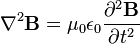$\nabla^2 \mathbf{B} = \mu_0 \epsilon_0 \frac{\partial^2 \mathbf{B}}{\partial t^2}$.

These differential equations are equivalent to the wave equation: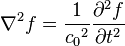$\nabla^2 f = \frac{1}{{c_0}^2} \frac{\partial^2 f}{\partial t^2} \,$
where
c0 is the speed of the wave in free space and
f describes a displacement

Or more simply:$\Box^2 f = 0$
where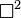$\Box^2$ is d'Alembertian: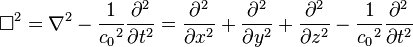$\Box^2 = \nabla^2 - \frac{1}{{c_0}^2} \frac{\partial^2}{\partial t^2} = \frac{\partial^2}{\partial x^2} + \frac{\partial^2}{\partial y^2} + \frac{\partial^2}{\partial z^2} - \frac{1}{{c_0}^2} \frac{\partial^2}{\partial t^2} \$

Notice that in the case of the electric and magnetic fields, the speed is: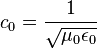$c_0 = \frac{1}{\sqrt{\mu_0 \epsilon_0}}$

Which, as it turns out, is the speed of light in free space. Maxwell's equations have unified the permittivity of free space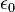$\epsilon_0$, the permeability of free space$\mu_0$, and the speed of light itself, c0. Before this derivation it was not known that there was such a strong relationship between light and electricity and magnetism.

But these are only two equations and we started with four, so there is still more information pertaining to these waves hidden within Maxwell's equations. Let's consider a generic vector wave for the electric field.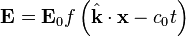$\mathbf{E} = \mathbf{E}_0 f\left( \hat{\mathbf{k}} \cdot \mathbf{x} - c_0 t \right)$

Here$\mathbf{E}_0$ is the constant amplitude,$f$ is any second differentiable function,$\hat{\mathbf{k}}$ is a unit vector in the direction of propagation, and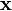${\mathbf{x}}$is a position vector. We observe that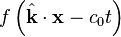$f\left( \hat{\mathbf{k}} \cdot \mathbf{x} - c_0 t \right)$ is a generic solution to the wave equation. In other words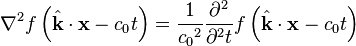$\nabla^2 f\left( \hat{\mathbf{k}} \cdot \mathbf{x} - c_0 t \right) = \frac{1}{{c_0}^2} \frac{\partial^2}{\partial^2 t} f\left( \hat{\mathbf{k}} \cdot \mathbf{x} - c_0 t \right)$,

for a generic wave traveling in the$\hat{\mathbf{k}}$ direction.

This form will satisfy the wave equation, but will it satisfy all of Maxwell's equations, and with what corresponding magnetic field?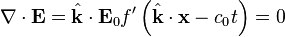$\nabla \cdot \mathbf{E} = \hat{\mathbf{k}} \cdot \mathbf{E}_0 f'\left( \hat{\mathbf{k}} \cdot \mathbf{x} - c_0 t \right) = 0$$\mathbf{E} \cdot \hat{\mathbf{k}} = 0$

The first of Maxwell's equations implies that electric field is orthogonal to the direction the wave propagates.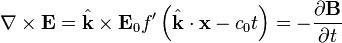$\nabla \times \mathbf{E} = \hat{\mathbf{k}} \times \mathbf{E}_0 f'\left( \hat{\mathbf{k}} \cdot \mathbf{x} - c_0 t \right) = -\frac{\partial \mathbf{B}}{\partial t}$$\mathbf{B} = \frac{1}{c_0} \hat{\mathbf{k}} \times \mathbf{E}$

The second of Maxwell's equations yields the magnetic field. The remaining equations will be satisfied by this choice of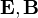$\mathbf{E},\mathbf{B}$.

Not only are the electric and magnetic field waves traveling at the speed of light, but they have a special restricted orientation and proportional magnitudes,$E_0 = c_0 B_0$, which can be seen immediately from the Poynting vector. The electric field, magnetic field, and direction of wave propagation are all orthogonal, and the wave propagates in the same direction as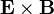$\mathbf{E} \times \mathbf{B}$.

From the viewpoint of an electromagnetic wave traveling forward, the electric field might be oscillating up and down, while the magnetic field oscillates right and left; but this picture can be rotated with the electric field oscillating right and left and the magnetic field oscillating down and up. This is a different solution that is traveling in the same direction. This arbitrariness in the orientation with respect to propagation direction is known as polarization.